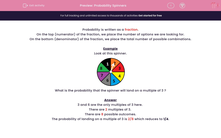# Probability Spinners

In this worksheet, students work out the probabilities of getting the given results on a spinner.Key stage:  KS 2

Curriculum topic:   Maths and Numerical Reasoning

Curriculum subtopic:   Probability

Difficulty level:#### Worksheet Overview

Probability is written as a fraction.

On the top (numerator) of the fraction, we place the number of options we are looking for.

On the bottom (denominator) of the fraction, we place the total number of possible combinations.

Example

Look at this spinner.What is the probability that the spinner will land on a multiple of 3 ?

3 and 6 are the only multiples of 3 here.

There are 2 multiples of 3.

There are 8 possible outcomes.

The probability of landing on a multiple of 3 is 2/8 which reduces to 1/4.

### What is EdPlace?

We're your National Curriculum aligned online education content provider helping each child succeed in English, maths and science from year 1 to GCSE. With an EdPlace account you’ll be able to track and measure progress, helping each child achieve their best. We build confidence and attainment by personalising each child’s learning at a level that suits them.

Get started